# Data sufficiency Quiz for RRB PO & RRB CLERK : Quiz – 6

## Data sufficiency Quiz for RRB PO & RRB CLERK : Quiz – 6

Data Sufficiency plays a significant role in Quantitative Aptitude Section of banking exams such as IBPS, SBI and RBI PO and Clerk. You will get at least 4-5 questions from Data Sufficiency in one of IBPS, SBI and RBI PO & clerk exam. So, aspirants should focus on Data Sufficiency questions in detail. Here, we are providing you with the Data Sufficiency questions quiz with the detailed solution so that you can easily prepare for Data Sufficiency questions. We are providing here all-important latest pattern-based questions and Previous Year Questions of Data Sufficiency of various Government Exam like IBPS, SBI, and RBI PO and Clerk exam. This Data Sufficiency quiz we are providing is free. Attempt this Data Sufficiency quiz to practice important questions with answers and solutions. And score better in IBPS, SBI and RBI PO and Clerk exam.

Data Sufficiency Quiz to improve your Quantitative Aptitude for SBI Po & SBI clerk exam, IBPS PO & IBPS Clerk exam, IBPS RRB PO and assistant exam, LIC AAO, LIC Assistant and other competitive exam.

Directions (1 – 10): Each question below is followed by two statements [I] or A, and [II] or B. You have to determine whether the data given in the statements are sufficient for answering the question. You should use the data and your knowledge of Mathematics to choose between the possible answers. Read the statements and Answer.
1. In how many days 8 women can complete the work?
[I]. 10 men & 12 Women can finish the work in 10/7days.
[II]. 5 Men and 6 women can finish the work in 20/7 days.
(a) if the Statement [I] alone is sufficient to answer the question but the Statement [II] alone is not sufficient
(b) if the Statement [II] alone is sufficient to answer the question but the Statement [I] alone is not sufficient
(c) if both Statement [I] and [II] together are needed to answer the question
(d) if either the Statement [I] alone or Statement [II] alone is sufficient to answer the question
(e) if you cannot get the answer from the Statements [I] and [II] together but need even more data2. What is the radius of the circle?
[I]. Length of a rectangle is 10% more than the breadth. Area of rectangle is 440 cm². Length of rectangle equals to circumference of circle.
[II] .Circumference of circle is equal to side of square having area 484 cm²
(a) if the Statement [I] alone is sufficient to answer the question but the Statement [II] alone is not sufficient
(b) if the Statement [II] alone is sufficient to answer the question but the Statement [I] alone is not sufficient
(c) if both Statement [I] and [II] together are needed to answer the question
(d) if either the Statement [I] alone or Statement [II] alone is sufficient to answer the question
(e) if you cannot get the answer from the Statements [I] and [II] together but need even more data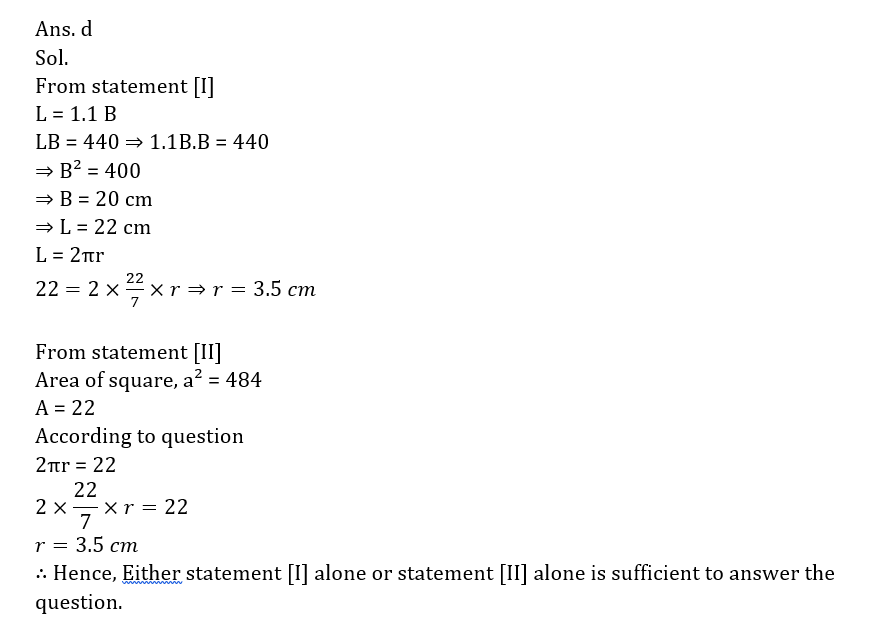3. Ram sold an item. Find the M.P of the item?
[I]. Ram gave two successive discounts is 20% & 5% on marked price. But after that take 25% more on discounted price as tax. Ram can earn 40 more If he sell the item at MP.
[II] .Ram gave two successive discount of MP i.e., 15% & 20% whereas Ram kept M.P. 50% more than the C.P. of that item.
(a) if the Statement [I] alone is sufficient to answer the question but the Statement [II] alone is not sufficient
(b) if the Statement [II] alone is sufficient to answer the question but the Statement [I] alone is not sufficient
(c) if both Statement [I] and [II] together are needed to answer the question
(d) if either the Statement [I] alone or Statement [II] alone is sufficient to answer the question
(e) if you cannot get the answer from the Statements [I] and [II] together but need even more data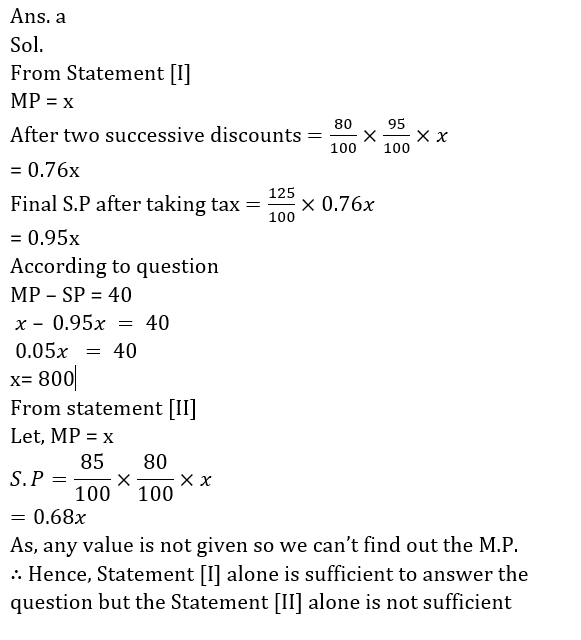4. Find the amount invested by Jagriti?
[I]. If jagriti invested half of the amount in Yes Bank at 5% for 3years and half the amount in Kotak Bank at 6% for 5 year, she got Rs 4500 as Simple Interest.
[II]. Jagriti will get 2420 more if she will invest in a bank at 10% p.a. for 3 year at compound interest rather than 10% p.a. for 2 year in same bank at compound interest
(a) if the Statement [I] alone is sufficient to answer the question but the Statement [II] alone is not sufficient
(b) if the Statement [II] alone is sufficient to answer the question but the Statement [I] alone is not sufficient
(c) if both Statement [I] and [II] together are needed to answer the question
(d) if either the Statement [I] alone or Statement [II] alone is sufficient to answer the question
(e) if you cannot get the answer from the Statements [I] and [II] together but need even more data5. What is the weight of Raju?
[I]. There are four person Raju, Ramesh, Rajan, Rajguru. Average of weight of these four is 51.25. Average weight of Rajan and Rajguru is 47.5. Average weight of Ramesh & Rajguru is 57.5.
[II]. In a class of 50 students having 30 kg as average weight If one students of weight 30 kg is replaced by Rajan then the average increases by 0.4.
(a) if the Statement [I] alone is sufficient to answer the question but the Statement [II] alone is not sufficient
(b) if the Statement [II] alone is sufficient to answer the question but the Statement [I] alone is not sufficient
(c) if both Statement [I] and [II] together are needed to answer the question
(d) if either the Statement [I] alone or Statement [II] alone is sufficient to answer the question
(e) if you cannot get the answer from the Statements [I] and [II] together but need even more data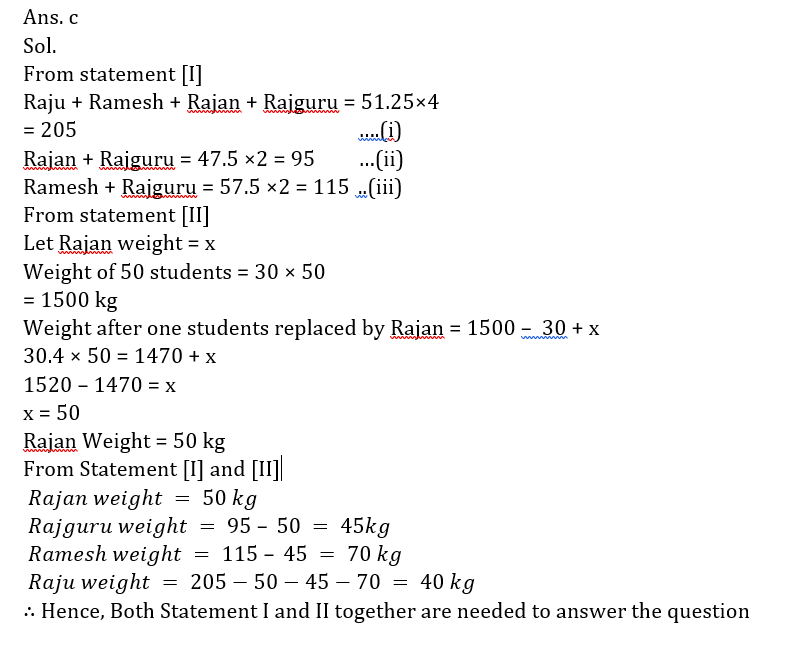6. The ages of Pradumn and Gunit are in the ratio of 7 : 5. What is the age of Pradumn ?
A. The ages of Pradumn and Nandini are in the ratio of 3 : 1.
B. After 7 years the ratio of Pradumn’s and Aviral’s age will be 4 : 3.
(a)if the statement A alone is sufficient to answer the question, but the statement B alone is not sufficient
(b) if the statement B alone is sufficient to answer the question, but the statement A alone is not sufficient
(c)if both statement A and B together are needed to answer the question
(d) if either the statement A alone or statement B alone is sufficient to answer the question
(e) If you cannot get the answer from the statements A and B together, but need even more data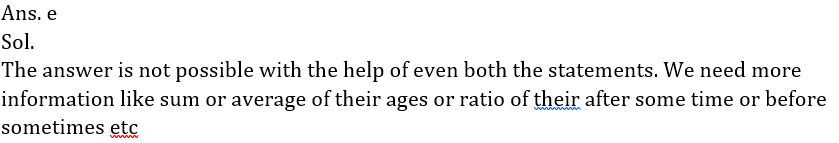7. What is the salary of B, in a group of A, B, C and D whose average salary is Rs. 62,880 ?
A. Total of the salary of A and C is exact multiple of 8.
B. Average of the salary of A, C and D isRs. 61,665.
(a)if the statement A alone is sufficient to answer the question, but the statement B alone is not sufficient
(b) if the statement B alone is sufficient to answer the question, but the statement A alone is not sufficient
(c)if both statement A and B together are needed to answer the question
(d) if either the statement A alone or statement B alone is sufficient to answer the question
(e) If you cannot get the answer from the statements A and B together, but need even more data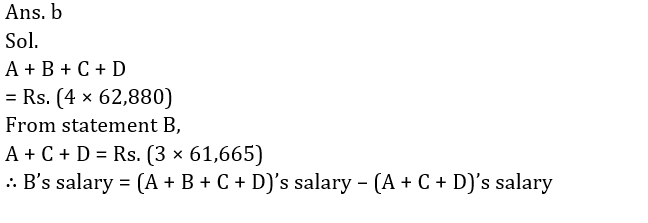8. What is the profit earned by selling a printer for Rs. 3,000 ?
A. The cost price of 6 such printers is equal to selling price of 5 such printers.
B. 20% profit is earned by selling each printer.
(a)if the statement A alone is sufficient to answer the question, but the statement B alone is not sufficient
(b) if the statement B alone is sufficient to answer the question, but the statement A alone is not sufficient
(c)if both statement A and B together are needed to answer the question
(d) if either the statement A alone or statement B alone is sufficient to answer the question
(e) If you cannot get the answer from the statements A and B together, but need even more data9. What is the ratio of the total number of girls to the total number of boys in the school ?
A. The ratio of the total number of boys to the total number of girls, last year was 4 : 5.
B. There are 3500 students in the school out of which 60% are boys.
(a)if the statement A alone is sufficient to answer the question, but the statement B alone is not sufficient
(b) if the statement B alone is sufficient to answer the question, but the statement A alone is not sufficient
(c)if both statement A and B together are needed to answer the question
(d) if either the statement A alone or statement B alone is sufficient to answer the question
(e) If you cannot get the answer from the statements A and B together, but need even more data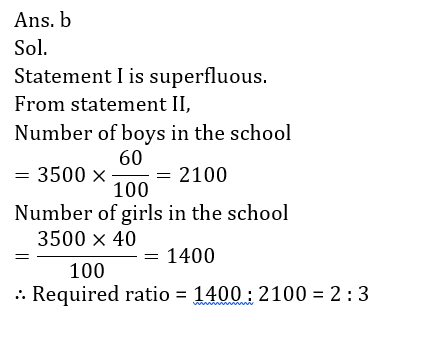10. What is the rate of interest p.c.p.a. on an amount of Rs. 12,000 deposited in a Bank ?
A. The difference between the simple interest and compound interest is Rs. 172.8.
B. The simple interest for two years is Rs. 2,880
(a)if the statement A alone is sufficient to answer the question, but the statement B alone is not sufficient
(b) if the statement B alone is sufficient to answer the question, but the statement A alone is not sufficient
(c)if both statement A and B together are needed to answer the question
(d) if either the statement A alone or statement B alone is sufficient to answer the question
(e) If you cannot get the answer from the statements A and B together, but need even more data#### ttempt Quantitative Aptitude Topic Wise Online Test Series

Recommended PDF’s for 2021:

### 2021 Preparation Kit PDF

#### Most important PDF’s for Bank, SSC, Railway and Other Government Exam : Download PDF Now

AATMA-NIRBHAR Series- Static GK/Awareness Practice Ebook PDF Get PDF here
The Banking Awareness 500 MCQs E-book| Bilingual (Hindi + English) Get PDF here
AATMA-NIRBHAR Series- Banking Awareness Practice Ebook PDF Get PDF here
Computer Awareness Capsule 2.O Get PDF here
AATMA-NIRBHAR Series Quantitative Aptitude Topic-Wise PDF 2020 Get PDF here
Memory Based Puzzle E-book | 2016-19 Exams Covered Get PDF here
Caselet Data Interpretation 200 Questions Get PDF here
Puzzle & Seating Arrangement E-Book for BANK PO MAINS (Vol-1) Get PDF here
ARITHMETIC DATA INTERPRETATION 2.O E-book Get PDF here
3